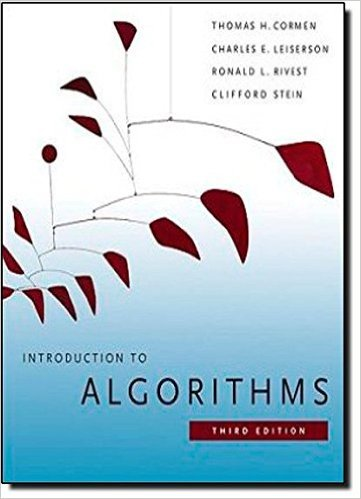×
Get Full Access to Introduction To Algorithms - 3 Edition - Chapter 4 - Problem 4-5
Get Full Access to Introduction To Algorithms - 3 Edition - Chapter 4 - Problem 4-5

×

# Chip testing Professor Diogenes has n supposedly identicalISBN: 9780262033848 130

## Solution for problem 4-5 Chapter 4

Introduction to Algorithms | 3rd Edition

• Textbook Solutions
• 2901 Step-by-step solutions solved by professors and subject experts
• Get 24/7 help from StudySoup virtual teaching assistantsIntroduction to Algorithms | 3rd Edition

4 5 1 349 Reviews
13
1
Problem 4-5

Step-by-Step Solution:
Step 1 of 3

Calculus 3 EX . Show that the equation ×2+y2+ -22-6×22=11 . 4y a sphere . Find center and radius if so . we around gives want move = ( X -a)2 + which is ' . / (X-3) + ( yt2)2t 1Z -1)2 . 25 a Sphere W/ center (3 , -2,1 ) radius 5 a square yes , c+2-2-22-+1 thy2t446×+9e+! ×2+bx+(b㱺 _ (b㱺2 3-

Step 2 of 3

Step 3 of 3